Author: Oscar Cronquist Article last updated on January 22, 2018

I have built a sheet to track time at work. It is very simple, there are 13 sheets, one for each month and a summary sheet.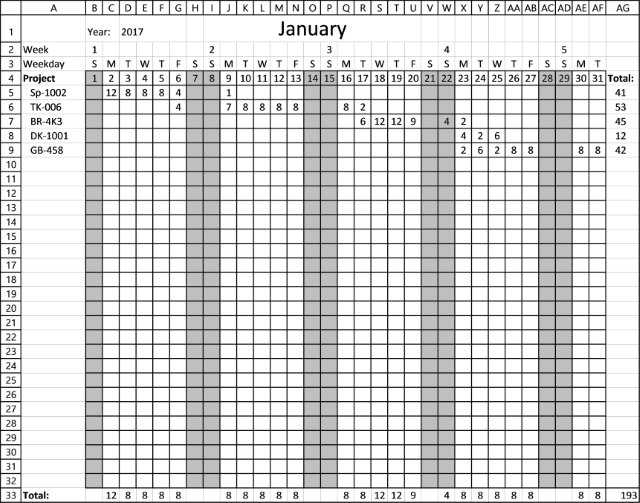The above picture shows you January 2017, simply enter the project name in column A and the hours in cell range B5:AF32. Row 4 contains dates for January, row 3 the weekdays and row 2 the week numbers. Saturday and Sundays are colored grey.

The Summary sheet, see picture below, contains all projects entered in all month sheets and a total, both for the month and project. A quite large array formula extracts all project names in column B, you don't need to do that manually.

A simple SUMIF function sums the values from each monthly sheet, excel takes care of that too. There is no vba in this workbook, you can find the download link below.

If you change the year on sheet "Summary" you will notice that the monthly sheets change accordingly, you don't need to change weekdays, week number or color weekends on each sheet, excel will do it for you.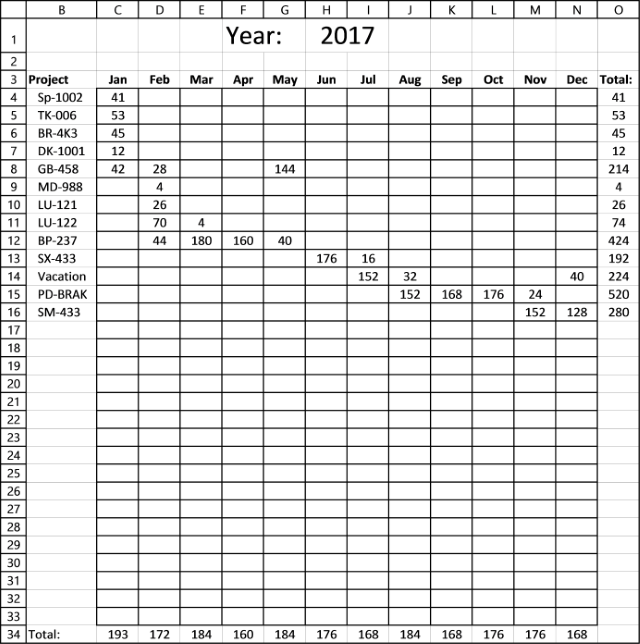Any suggestions for improvement?

Work time trackerv2.xlsx

### How I built this time tracker

The workbook has a summary sheet and sheets for each month in a year. The summary sheet allows you to enter the year in cell H1, I also changed the font size for that cell.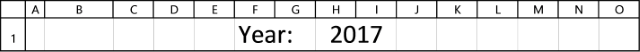#### Building monthly sheets

The first monthly sheet is January, this sheet will be the template for the remaining months. This means I will copy this sheet and change the date for each sheet.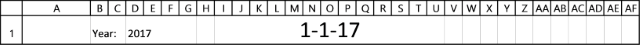Cell D1 contains this formula: =Summary!H1
This lets you change the year on the summary sheet and all other monthly sheets will be instantly updated.

Formula in cell I1: =DATE(D1,1,1)
Select cell I1 and press CTRL + 1 to open the formatting cells dialog box. Change formatting category to "Custom".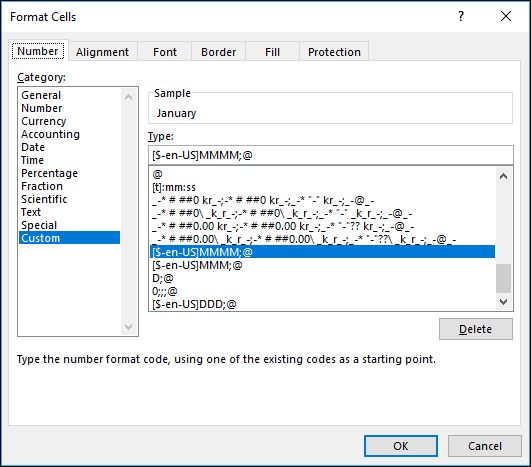Change Type to MMMM;@
If this is not working you need to find out your regional settings in Windows and change MMMM accordingly. This web page from Microsoft explains how to use the formatting dialog box.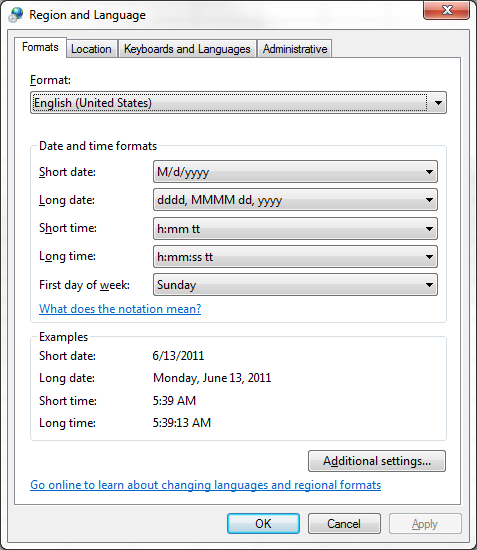This is what cell I1 now looks like:Time to add dates,type 1 to 31 in cell range B4:AH4. I also change the cell width to 21 pixels. Type "Project" in cell A4, cells below contains project names.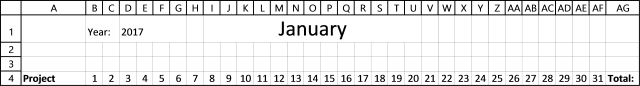Add text "Total:" to cell AG4 and use this formula in cell AG5:=SUM(B5:AF5)
Copy cell AG5 and paste to cell range AG6:AG32. See picture below.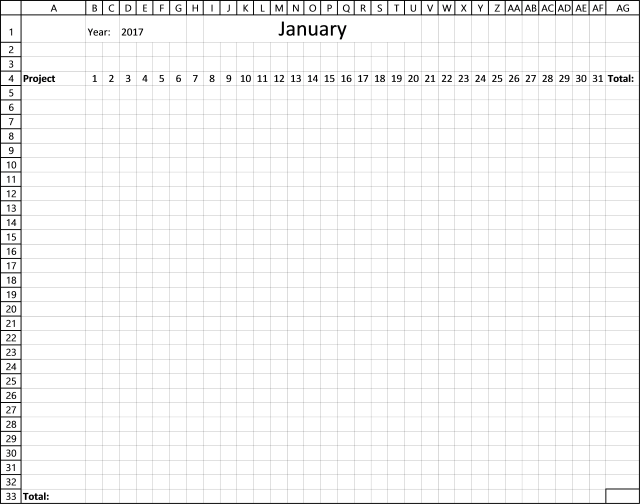Add text "Total:" to cell A33 and use this formula in cell B33:=SUM(B5:B32)
Copy cell A33 and paste to cell range B33:AF33. See picture above.

To make this sheet easier to read I want the row above dates to contain the abbreviation of days of the week. I am using this formula in cell B3:

=IF(MONTH(\$B\$4)=MONTH(B4), CHOOSE(WEEKDAY(B4,2),"M","T","W","T","F","S","S"),""). See picture below.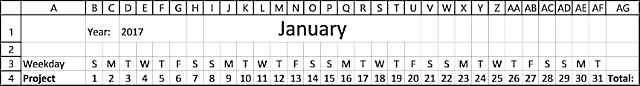I also want the row above days of the week to contain the week number. Formula in cell B2:

=WEEKNUM(B4,1)

But I only want that number above a date when a new week starts.

Formula in cell C2:

=IF((WEEKNUM(C4,1)<>WEEKNUM(B4,1))*(MONTH(\$B\$4)=MONTH(B4)), WEEKNUM(C4,1),"")

Copy cell C2 and paste to D2:AF2. See pic below.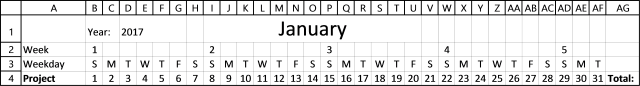The following conditional formatting formula highlights weekends gray:

(WEEKDAY(B\$4,2)>5)*(MONTH(B\$4)=MONTH(\$B\$4))

1. Select cell range B4:AF32
2. Go to tab Home on the ribbon
3. Click "Conditional Formatting" button and then click "New Rule.."
4. Click "Use a formula to determine which cells to format"
5. Paste above formula to field "Format values where this formula is true:"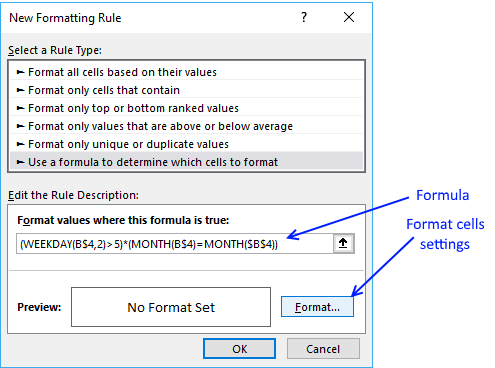6. Click "Format" button
7. Go to tab "Fill"
8. Pick a color9. Click OK button

Worksheet "January" now looks like this: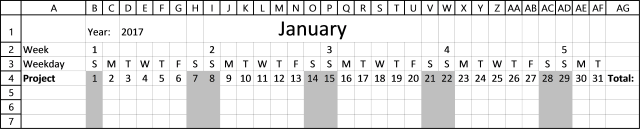The conditional formatting changes depending what month and year it is, this is not something you have to manually do.

Next thing is the grid pattern, here is the conditional formatting formula:

=IF(\$AI\$3="Off",,MONTH(B\$4)=MONTH(\$B\$4))

To build formatting formula, repeat above steps 1 to 8 except instead of picking a color, go to tab "Border" and click "Outline, then click OK button. See picture below.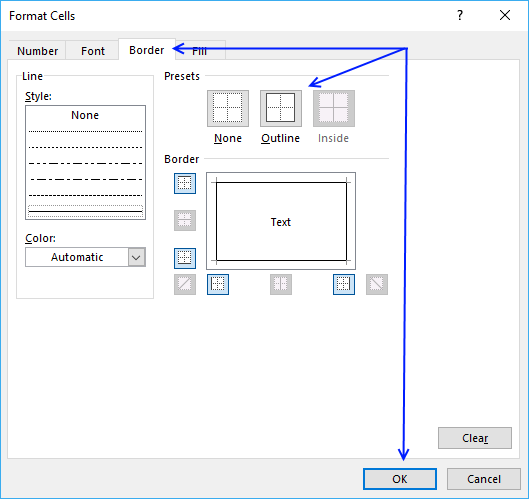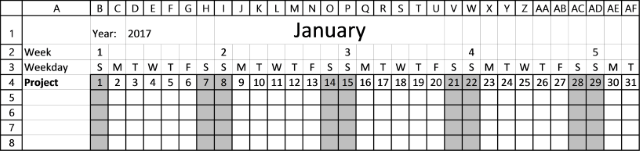Worksheet January is now ready, time to copy the worksheet and create worksheets for the remaining months.

1. Right click on sheet "January"
2. Click "Move or Copy..."
3. Select "move to end" and "Create a copy"
4. Click Ok button

Repeat above steps until you have a worksheet for each month in a year.

1. Rename the sheet after January to February
2. Change formula in cell I1 to =DATE(D1,2,1)
Note that February is the second month in a year and the second argument in the formula is then 2.

Repeat steps 1 and 2 above for all remaining worksheets.